Static Electricity - Lesson 1 - Basic Terminology and Concepts

# Charge Interactions

Suppose that you rubbed a balloon with a sample of animal fur such as a wool sweater or even your own hair. The balloon would likely become charged and its charge would exert a strange influence upon other objects in its vicinity. If some small bits of paper were placed upon a table and the balloon were brought near and held above the paper bits, then the presence of the charged balloon might create a sufficient attraction for the paper bits to raise them off the table. This influence - known as an electric force - occurs even when the charged balloon is held some distance away from the paper bits. The electric force is a non-contact force. Any charged object can exert this force upon other objects - both charged and uncharged objects. One goal of this unit of The Physics Classroom is to understand the nature of the electric force. In this part of Lesson 1, two simple and fundamental statements will be made and explained about the nature of the electric force.

Perhaps you have heard it said so many times that it sounds like a cliché.

Opposites attract. And likes repel.

These two fundamental principles of charge interactions will be used throughout the unit to explain the vast array of static electricity phenomena. As mentioned in the previous section of Lesson 1, there are two types of electrically charged objects - those that contain more protons than electrons and are said to be positively charged and those that contain less protons than electrons and are said to be negatively charged. These two types of electrical charges - positive and negative - are said to be opposite types of charge. And consistent with our fundamental principle of charge interaction, a positively charged object will attract a negatively charged object. Oppositely charged objects will exert an attractive influence upon each other. In contrast to the attractive force between two objects with opposite charges, two objects that are of like charge will repel each other. That is, a positively charged object will exert a repulsive force upon a second positively charged object. This repulsive force will push the two objects apart. Similarly, a negatively charged object will exert a repulsive force upon a second negatively charged object. Objects with like charge repel each other.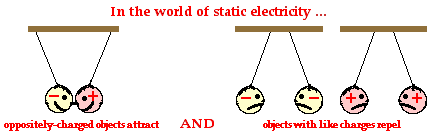### The Electric Force and Newton's Third Law

This electric force exerted between two oppositely charged objects or two like charged objects is a force in the same sense that friction, tension, gravity and air resistance are forces. And being a force, the same laws and principles that describe any force describe the electrical force. One of those laws was Newton's law of action-reaction (discussed in Unit 2 of The Physics Classroom). According to Newton's third law, a force is simply a mutual interaction between two objects that results in an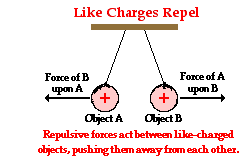equal and opposite push or pull upon those objects. Let's apply Newton's third law to describe the interaction between Object A and Object B, both having positive charge.

Object A exerts a rightward push upon Object B. Object B exerts a leftward push upon Object A. See diagram at right. These two pushing forces have equal magnitudes and are exerted in opposite directions of each other. Each object does its own pushing upon the other. The push upon Object B (by Object A) is directed away from Object A; and the push upon Object A (by Object B) is directed away from Object B. Because of the away from nature of the mutual interaction, the force is said to be repulsive.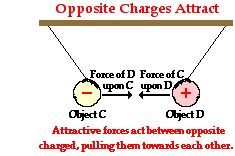Now let's apply the same action-reaction principle to two oppositely charged objects - Object C (positive) and Object D (negative). See diagram at right. Object C exerts a leftward pull upon object D. Object D exerts a rightward pull upon Object C. Again, each object does its own pulling of the other. Just as before, these two forces have equal magnitudes and are exerted in opposite directions of each other. However in this instance, the direction of the force on Object D is towards Object C and the direction of the force on Object C is towards object D. Because of the towards each other nature of the mutual interaction, the force is described as being attractive.

### Interaction Between Charged and Neutral Objects

The interaction between two like-charged objects is repulsive. The interaction between two oppositely charged objects is attractive. What type of interaction is observed between a charged object and a neutral object? The answer is quite surprising to many students of physics. Any charged object - whether positively charged or negatively charged - will have an attractive interaction with a neutral object. Positively charged objects and neutral objects attract each other; and negatively charged objects and neutral objects attract each other.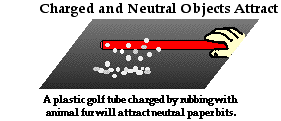This third interaction between charged and neutral objects is often demonstrated by physics teachers or experienced by students in physics lab activities. For instance, if a charged balloon is held above neutral bits of paper, the force of attraction for the paper bits will be strong enough to overwhelm the downward force of gravity and raise the bits of paper off the table. If a charged plastic tube is held above some bits of paper, the tube will exert an attractive influence upon the paper to raise it off the table. And to the bewilderment of many, a charged rubber balloon can be attracted to a wooden cabinet with enough force that it sticks to the cabinet. Any charged object - plastic, rubber, or aluminum - will exert an attractive force upon a neutral object. And in accordance with Newton's law of action-reaction, the neutral object attracts the charged object.

### Flickr Physics Photo

A balloon is charged by rubbing it with hair. It is then brought near some bits of paper. The charged balloon attracts the paper bits, lifting them up off the table. This demonstrates the attraction between charged objects and neutral objects.### Repulsion versus Attraction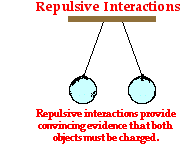Because charged objects interact with their surroundings, an observed interaction provides possible evidence that an object is charged. Suppose that you enter the physics classroom and observe two balloons suspended from the ceiling. Rather than hanging straight down vertically, the balloons are hanging at an angle, exhibiting a repulsive interaction as shown at the right. The only way that two objects can repel each other is if they are both charged with the same type of charge. Thus, the repulsion of the balloons provides conclusive evidence that both balloons are charged and charged with the same type of charge. One could not conclude that the balloons are both positively charged or both negatively charged. Additional information or further testing would be required to make a conclusion about the type of excess charge present upon the balloons. Nonetheless, one can be convinced that both balloons possess an excess charge - either positive or negative.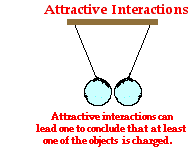Now let's contrast the observation of repulsion with that of attraction. Suppose that you now enter the physics classroom and observe two balloons suspended from the ceiling and exhibiting an attractive interaction as shown at the right. There are two underlying reasons for two objects attracting each other. One balloon could be neutral and the other balloon charged or both balloons could be charged with the opposite type of charge. Thus, your only conclusion could be that at least one of the balloons is charged. The other balloon is either neutral or charged with the opposite type of charge. You cannot draw a conclusion about which one of the balloons is charged or what type of charge (positive or negative) the charged balloon possesses. Additional information or further testing would be required to make these conclusions. For example, if you could take each balloon and individually bring them near some neutral bits of paper, you could test to see if each individual balloon is charged or neutral. If a balloon were charged, then it would exhibit an attractive interaction with the neutral paper bits. On the other hand, an uncharged balloon would not interact at all with neutral paper bits.

The above thought experiments illustrate the conclusive nature of a repulsive interaction. When objects repel each other, one can be certain that both objects are charged. On the other had, the observation of an attractive interaction leads to limited conclusions. At best, one can conclude that at least one of the objects is charged.

We'll conclude this part of Lesson 1 by asking the question "How can a charged object and a neutral object attract?" As you've read this page, you might have been thinking something like "But I've only heard of two fundamental charge interactions - opposites attract and likes repel. Where did this third charge interaction come from?"

In all likelihood, most of us have only heard of two types of charge interactions (opposites attract and likes repel); and both of these charge interactions are fundamental interactions. The third statement - any charged object and a neutral object will attract each other - is simply an observable fact that can be explained by the two fundamental charge interactions. How? The explanation of this third charge interaction will be saved for the last page of Lesson 1. But first, the subject of conductors and insulators must be explored in order to understand our third type of charge interaction.

### Flickr Physics Photo

Two like-charged balloons hang from a common point from the ceiling. The repulsion effects cause them to hang at an angle from their usual vertical alignment. A plastic tube is charged by rubbing with synthetic fur. The plastic tube is inserted into the space between the balloons, causing even further repulsion.### We Would Like to Suggest ...Sometimes it isn't enough to just read about it. You have to interact with it! And that's exactly what you do when you use one of The Physics Classroom's Interactives. We would like to suggest that you combine the reading of this page with the use of our Charging Interactive. You can find it in the Physics Interactives section of our website. The Charging Interactive is an electrostatics "playground" that allows a learner to investigate a variety of concepts related to charge, charge interactions, charging processes, and grounding. Once you get the hang of the concepts, put your game-face on tap the Play button.

Use your understanding of charge to answer the following questions. When finished, click the button to view the answers.

1. Electrical forces ____.

a. can cause objects to only attract each other

b. can cause objects to only repel each other

c. can cause objects to attract or repel each other

d. have no effect on objects

2. On two occasions, the following charge interactions between balloons A, B and C are observed. In each case, it is known that balloon B is charged negatively. Based on these observations, what can you conclusively confirm about the charge on balloon A and C for each situation.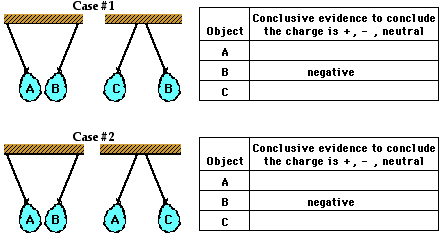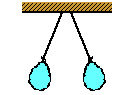3. Upon entering the room, you observe two balloons suspended from the ceiling. You notice that instead of hanging straight down vertically, the balloons seems to be repelling each other. You can conclusively say ...

a. both balloons have a negative charge.

b. both balloons have a positive charge.

c. one balloon is charge positively and the other negatively.

d. both balloons are charged with the same type of charge.

4. Jean Yuss is investigating the charge on several objects and makes the following findings.

 Object C Object D Object E Object F attracts B repels C attracts D repels F attracts A

Jean knows that object A is negatively charged and object B is electrically neutral. What can Jean Yuss definitively conclude about the charge on objects C, D, E, and F? Explain.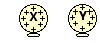6. Two objects are charged as shown at the right. Object X will ____ object Y.

 a. attract b. repel c. not affect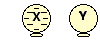7. Two objects are shown at the right. One is neutral and the other is negative. Object X will ____ object Y.

 a. attract b. repel c. not affect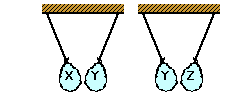8. Balloons X , Y and Z are suspended from strings as shown at the right. Negatively charged balloon X attracts balloon Y and balloon Y attracts balloon Z. Balloon Z ____. List all that apply.

a. may be positively charged

b. may be negatively charged

c. may be neutral

d. must be positively charged

e. must be negatively charged

f. must be neutral

(NOTE: This is an exercise in logic and reasoning as much as it is an exercise in physics.)

Next Section: